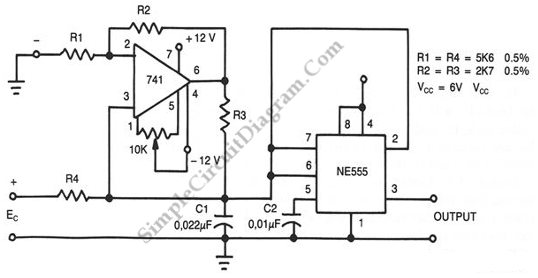# Voltage To Frequency Converter

Using this circuit, we can accept positive or negative or differential control voltages. When the control voltage is zero, the output frequency is zero. To charge the timing capacitor C1 linearly, the 741 op amp forms a current source controlled by the voltage EC. The capacitor charges and discharges between 1/3 Vcc and 2/3 Vcc because NE555 is connected in the astable mode. 10K potentiometer is adjust the offset so that the frequency is zero when the input is zero. For component values shown f≈4.2 Ec kHz, the output frequency will be proportional to the difference between the two voltages if two dc voltages are applied to the ends of R1 and R4.[circuit’s schematic diagram source: seekic.com]